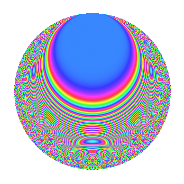# Properties

 Label 1335.2.aLevel 1335 Weight 2 Character orbit a Rep. character $$\chi_{1335}(1,\cdot)$$ Character field $$\Q$$ Dimension 59 Newforms 11 Sturm bound 360 Trace bound 2

# Related objects

## Defining parameters

 Level: $$N$$ = $$1335 = 3 \cdot 5 \cdot 89$$ Weight: $$k$$ = $$2$$ Character orbit: $$[\chi]$$ = 1335.a (trivial) Character field: $$\Q$$ Newforms: $$11$$ Sturm bound: $$360$$ Trace bound: $$2$$ Distinguishing $$T_p$$: $$2$$

## Dimensions

The following table gives the dimensions of various subspaces of $$M_{2}(\Gamma_0(1335))$$.

Total New Old
Modular forms 184 59 125
Cusp forms 177 59 118
Eisenstein series 7 0 7

The following table gives the dimensions of the cuspidal new subspaces with specified eigenvalues for the Atkin-Lehner operators and the Fricke involution.

$$3$$$$5$$$$89$$FrickeDim.
$$+$$$$+$$$$+$$$$+$$$$6$$
$$+$$$$+$$$$-$$$$-$$$$9$$
$$+$$$$-$$$$+$$$$-$$$$10$$
$$+$$$$-$$$$-$$$$+$$$$3$$
$$-$$$$+$$$$+$$$$-$$$$11$$
$$-$$$$+$$$$-$$$$+$$$$4$$
$$-$$$$-$$$$+$$$$+$$$$3$$
$$-$$$$-$$$$-$$$$-$$$$13$$
Plus space$$+$$$$16$$
Minus space$$-$$$$43$$

## Trace form

 $$59q$$ $$\mathstrut +\mathstrut 5q^{2}$$ $$\mathstrut +\mathstrut 3q^{3}$$ $$\mathstrut +\mathstrut 69q^{4}$$ $$\mathstrut -\mathstrut q^{5}$$ $$\mathstrut +\mathstrut q^{6}$$ $$\mathstrut +\mathstrut 8q^{7}$$ $$\mathstrut +\mathstrut 9q^{8}$$ $$\mathstrut +\mathstrut 59q^{9}$$ $$\mathstrut +\mathstrut O(q^{10})$$ $$59q$$ $$\mathstrut +\mathstrut 5q^{2}$$ $$\mathstrut +\mathstrut 3q^{3}$$ $$\mathstrut +\mathstrut 69q^{4}$$ $$\mathstrut -\mathstrut q^{5}$$ $$\mathstrut +\mathstrut q^{6}$$ $$\mathstrut +\mathstrut 8q^{7}$$ $$\mathstrut +\mathstrut 9q^{8}$$ $$\mathstrut +\mathstrut 59q^{9}$$ $$\mathstrut +\mathstrut q^{10}$$ $$\mathstrut +\mathstrut 12q^{11}$$ $$\mathstrut +\mathstrut 5q^{12}$$ $$\mathstrut +\mathstrut 18q^{13}$$ $$\mathstrut +\mathstrut 3q^{15}$$ $$\mathstrut +\mathstrut 77q^{16}$$ $$\mathstrut +\mathstrut 6q^{17}$$ $$\mathstrut +\mathstrut 5q^{18}$$ $$\mathstrut -\mathstrut 4q^{19}$$ $$\mathstrut -\mathstrut 7q^{20}$$ $$\mathstrut +\mathstrut 8q^{21}$$ $$\mathstrut -\mathstrut 4q^{22}$$ $$\mathstrut -\mathstrut 3q^{24}$$ $$\mathstrut +\mathstrut 59q^{25}$$ $$\mathstrut -\mathstrut 2q^{26}$$ $$\mathstrut +\mathstrut 3q^{27}$$ $$\mathstrut +\mathstrut 32q^{28}$$ $$\mathstrut +\mathstrut 26q^{29}$$ $$\mathstrut +\mathstrut q^{30}$$ $$\mathstrut +\mathstrut 8q^{31}$$ $$\mathstrut +\mathstrut 73q^{32}$$ $$\mathstrut -\mathstrut 4q^{33}$$ $$\mathstrut +\mathstrut 18q^{34}$$ $$\mathstrut +\mathstrut 69q^{36}$$ $$\mathstrut +\mathstrut 42q^{37}$$ $$\mathstrut +\mathstrut 52q^{38}$$ $$\mathstrut +\mathstrut 10q^{39}$$ $$\mathstrut -\mathstrut 3q^{40}$$ $$\mathstrut -\mathstrut 2q^{41}$$ $$\mathstrut -\mathstrut 8q^{42}$$ $$\mathstrut +\mathstrut 12q^{43}$$ $$\mathstrut +\mathstrut 36q^{44}$$ $$\mathstrut -\mathstrut q^{45}$$ $$\mathstrut +\mathstrut 16q^{46}$$ $$\mathstrut -\mathstrut 8q^{47}$$ $$\mathstrut -\mathstrut 3q^{48}$$ $$\mathstrut +\mathstrut 83q^{49}$$ $$\mathstrut +\mathstrut 5q^{50}$$ $$\mathstrut -\mathstrut 10q^{51}$$ $$\mathstrut +\mathstrut 38q^{52}$$ $$\mathstrut +\mathstrut 58q^{53}$$ $$\mathstrut +\mathstrut q^{54}$$ $$\mathstrut -\mathstrut 4q^{55}$$ $$\mathstrut -\mathstrut 8q^{56}$$ $$\mathstrut +\mathstrut 20q^{57}$$ $$\mathstrut -\mathstrut 50q^{58}$$ $$\mathstrut -\mathstrut 4q^{59}$$ $$\mathstrut +\mathstrut 5q^{60}$$ $$\mathstrut +\mathstrut 26q^{61}$$ $$\mathstrut -\mathstrut 8q^{62}$$ $$\mathstrut +\mathstrut 8q^{63}$$ $$\mathstrut +\mathstrut 69q^{64}$$ $$\mathstrut -\mathstrut 14q^{65}$$ $$\mathstrut -\mathstrut 4q^{66}$$ $$\mathstrut -\mathstrut 8q^{67}$$ $$\mathstrut -\mathstrut 6q^{68}$$ $$\mathstrut +\mathstrut 12q^{69}$$ $$\mathstrut +\mathstrut 8q^{70}$$ $$\mathstrut -\mathstrut 24q^{71}$$ $$\mathstrut +\mathstrut 9q^{72}$$ $$\mathstrut -\mathstrut 22q^{73}$$ $$\mathstrut +\mathstrut 6q^{74}$$ $$\mathstrut +\mathstrut 3q^{75}$$ $$\mathstrut -\mathstrut 36q^{76}$$ $$\mathstrut -\mathstrut 42q^{78}$$ $$\mathstrut +\mathstrut 20q^{79}$$ $$\mathstrut +\mathstrut q^{80}$$ $$\mathstrut +\mathstrut 59q^{81}$$ $$\mathstrut -\mathstrut 6q^{82}$$ $$\mathstrut -\mathstrut 20q^{83}$$ $$\mathstrut +\mathstrut 56q^{84}$$ $$\mathstrut +\mathstrut 6q^{85}$$ $$\mathstrut +\mathstrut 36q^{86}$$ $$\mathstrut -\mathstrut 26q^{87}$$ $$\mathstrut +\mathstrut 60q^{88}$$ $$\mathstrut -\mathstrut q^{89}$$ $$\mathstrut +\mathstrut q^{90}$$ $$\mathstrut -\mathstrut 48q^{91}$$ $$\mathstrut -\mathstrut 32q^{92}$$ $$\mathstrut +\mathstrut 8q^{93}$$ $$\mathstrut +\mathstrut 8q^{94}$$ $$\mathstrut -\mathstrut 4q^{95}$$ $$\mathstrut -\mathstrut 35q^{96}$$ $$\mathstrut -\mathstrut 14q^{97}$$ $$\mathstrut -\mathstrut 35q^{98}$$ $$\mathstrut +\mathstrut 12q^{99}$$ $$\mathstrut +\mathstrut O(q^{100})$$

## Decomposition of $$S_{2}^{\mathrm{new}}(\Gamma_0(1335))$$ into irreducible Hecke orbits

Label Dim. $$A$$ Field CM Traces A-L signs $q$-expansion
$$a_2$$ $$a_3$$ $$a_5$$ $$a_7$$ 3 5 89
1335.2.a.a $$1$$ $$10.660$$ $$\Q$$ None $$-1$$ $$1$$ $$1$$ $$0$$ $$-$$ $$-$$ $$-$$ $$q-q^{2}+q^{3}-q^{4}+q^{5}-q^{6}+3q^{8}+\cdots$$
1335.2.a.b $$1$$ $$10.660$$ $$\Q$$ None $$1$$ $$1$$ $$-1$$ $$4$$ $$-$$ $$+$$ $$+$$ $$q+q^{2}+q^{3}-q^{4}-q^{5}+q^{6}+4q^{7}+\cdots$$
1335.2.a.c $$2$$ $$10.660$$ $$\Q(\sqrt{17})$$ None $$-1$$ $$2$$ $$2$$ $$0$$ $$-$$ $$-$$ $$-$$ $$q-\beta q^{2}+q^{3}+(2+\beta )q^{4}+q^{5}-\beta q^{6}+\cdots$$
1335.2.a.d $$3$$ $$10.660$$ $$\Q(\zeta_{14})^+$$ None $$-2$$ $$3$$ $$3$$ $$-5$$ $$-$$ $$-$$ $$+$$ $$q+(-1-\beta _{2})q^{2}+q^{3}+(\beta _{1}+\beta _{2})q^{4}+\cdots$$
1335.2.a.e $$3$$ $$10.660$$ $$\Q(\zeta_{18})^+$$ None $$0$$ $$-3$$ $$3$$ $$3$$ $$+$$ $$-$$ $$-$$ $$q-\beta _{1}q^{2}-q^{3}+\beta _{2}q^{4}+q^{5}+\beta _{1}q^{6}+\cdots$$
1335.2.a.f $$4$$ $$10.660$$ 4.4.2777.1 None $$0$$ $$4$$ $$-4$$ $$-7$$ $$-$$ $$+$$ $$-$$ $$q+\beta _{2}q^{2}+q^{3}+(1-\beta _{3})q^{4}-q^{5}+\beta _{2}q^{6}+\cdots$$
1335.2.a.g $$6$$ $$10.660$$ 6.6.10407557.1 None $$-4$$ $$-6$$ $$-6$$ $$1$$ $$+$$ $$+$$ $$+$$ $$q+(-1-\beta _{2})q^{2}-q^{3}+(2+\beta _{2}+\beta _{3}+\cdots)q^{4}+\cdots$$
1335.2.a.h $$9$$ $$10.660$$ $$\mathbb{Q}[x]/(x^{9} - \cdots)$$ None $$5$$ $$-9$$ $$-9$$ $$-3$$ $$+$$ $$+$$ $$-$$ $$q+(1-\beta _{1})q^{2}-q^{3}+(2-\beta _{1}+\beta _{2})q^{4}+\cdots$$
1335.2.a.i $$10$$ $$10.660$$ $$\mathbb{Q}[x]/(x^{10} - \cdots)$$ None $$0$$ $$10$$ $$-10$$ $$9$$ $$-$$ $$+$$ $$+$$ $$q+\beta _{1}q^{2}+q^{3}+(2+\beta _{2})q^{4}-q^{5}+\beta _{1}q^{6}+\cdots$$
1335.2.a.j $$10$$ $$10.660$$ $$\mathbb{Q}[x]/(x^{10} - \cdots)$$ None $$1$$ $$-10$$ $$10$$ $$-1$$ $$+$$ $$-$$ $$+$$ $$q+\beta _{1}q^{2}-q^{3}+(1+\beta _{2})q^{4}+q^{5}-\beta _{1}q^{6}+\cdots$$
1335.2.a.k $$10$$ $$10.660$$ $$\mathbb{Q}[x]/(x^{10} - \cdots)$$ None $$6$$ $$10$$ $$10$$ $$7$$ $$-$$ $$-$$ $$-$$ $$q+(1-\beta _{1})q^{2}+q^{3}+(2-\beta _{1}+\beta _{2})q^{4}+\cdots$$

## Decomposition of $$S_{2}^{\mathrm{old}}(\Gamma_0(1335))$$ into lower level spaces

$$S_{2}^{\mathrm{old}}(\Gamma_0(1335)) \cong$$ $$S_{2}^{\mathrm{new}}(\Gamma_0(15))$$$$^{\oplus 2}$$$$\oplus$$$$S_{2}^{\mathrm{new}}(\Gamma_0(89))$$$$^{\oplus 4}$$$$\oplus$$$$S_{2}^{\mathrm{new}}(\Gamma_0(267))$$$$^{\oplus 2}$$$$\oplus$$$$S_{2}^{\mathrm{new}}(\Gamma_0(445))$$$$^{\oplus 2}$$